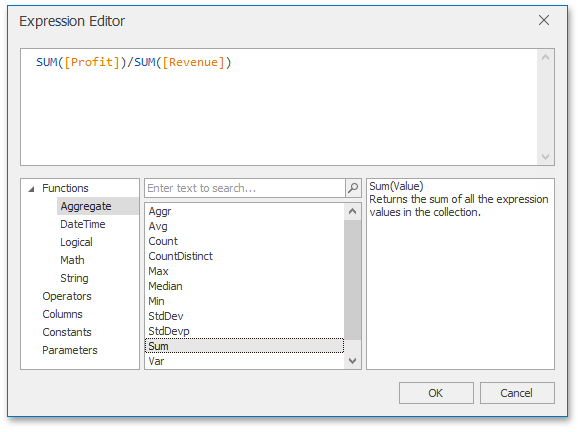20.1
19.2
19.1
18.2
18.1
17.2

# Summary Level Aggregations

The Dashboard Designer allows you to perform aggregations when constructing a calculated field expression. This allows you to evaluate calculated fields on a summary level.

In the Dashboard Designer, you can use the following set of predefined aggregate functions.Function

Description

Example

Avg(Value)

Returns the average of all the values in the expression.

Avg([Profit])

Count()

Returns the number of values.

Count()

CountNotNull(Value)

Returns a number of non-null objects in a collection.

CountNotNull([Orders])

CountDistinct(Value)

Returns the number of distinct values.

CountDistinct([Orders])

Max(Value)

Returns the maximum value across all records.

Max([Profit])

Min(Value)

Returns the minimum value across all records.

Min([Profit])

Mode(Value)

Returns the mode of the values.

Mode([Profit])

Median(Value)

Returns the median of the values.

Median([Profit])

Sum(Value)

Returns the sum of all values.

Sum([Profit])

Var(Value)

Returns an estimate of the variance of a population, where the sample is a subset of the entire population.

Var([Orders])

Varp(Value)

Returns the variance of a population, where the population is the entire data to be summarized.

Varp([Orders])

StdDev(Value)

Returns an estimate of the standard deviation of a population, where the sample is a subset of the entire population.

StdDev([Orders])

StdDevp(Value)

Returns the standard deviation of a population, where the population is the entire data to be summarized.

StdDevp([Orders])

These functions can be used for all types of numeric fields. After creating such calculated fields, you can use them as measures contained in an OLAP cube.

The following code snippet shows how to create a Margin calculated field based on the Profit and Revenue data source fields.

``````using DevExpress.DashboardCommon;
//...
CalculatedField margin = new CalculatedField("Sum([ProfitTarget]) / Sum([RevenueTarget])");
margin.DataType = CalculatedFieldType.Decimal;
margin.Name = "Margin";